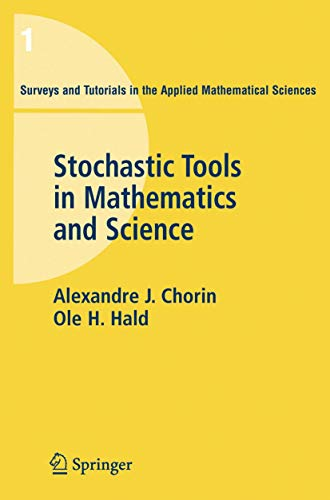# Stochastic Tools in Mathematics and Science (Surveys and Tutorials in the Applied Mathematical Sciences): v. 1

## Chorin, Alexandre J.; Hald, Ole H.

0 avg rating
( 0 ratings by Goodreads )View all copies of this ISBN edition:

Stochastic Tools in Mathematics and Science is an introductory book on probability-based modeling. It covers basic stochastic tools used in physics, chemistry, engineering and the life sciences. The topics covered include conditional expectations, stochastic processes, Brownian motion and its relation to partial differential equations, Langevin equations, the Liouville and Fokker-Planck equations, as well as Markov chain Monte Carlo algorithms, renormalization and dimensional reduction, and basic equilibrium and non-equilibrium statistical mechanics. The applications include data assimilation, prediction from partial data, spectral analysis, and turbulence. A noteworthy feature of the book is the systematic analysis of memory effects. The presentation is mathematically attractive, and should form a useful bridge between the theoretical treatments familiar to mathematical specialists and the more practical questions raised by specific applications. The book is based on lecture notes from a class that has attracted graduate and advanced undergraduate students from mathematics and from many other science departments at the University of California, Berkeley. Each chapter is followed by exercises. The book will be useful for scientists and engineers working in a wide range of fields and applications.

"synopsis" may belong to another edition of this title.

Review:

From the reviews of the first edition:

"The book is well-written and provides a very useful introduction to various areas of applications of stochastic processes." (Evelyn Buckwar, Zentralblatt MATH, Vol. 1086, 2006)

"The authors write ??? that this book is based on lecture notes for graduate courses on ???the stochastic methods of applied mathematics???. Their aim is ??? to show various applications of probability to mathematics students and, on the other hand, to introduce nonmathematical students to the general ideas of probabilistic methods. ??? This is an excellent concise textbook which can be used for self-study by graduate and advanced undergraduate students and as a recommended textbook for an introductory course on probabilistic tools in science." (Mikhail V. Tretyakov, Mathematical Reviews, Issue 2006 j)

From the reviews of the first edition:

"The book is well-written and provides a very useful introduction to various areas of applications of stochastic processes." (Evelyn Buckwar, Zentralblatt MATH, Vol. 1086, 2006)

"The authors write a ] that this book is based on lecture notes for graduate courses on a ~the stochastic methods of applied mathematicsa (TM). Their aim is a ] to show various applications of probability to mathematics students and, on the other hand, to introduce nonmathematical students to the general ideas of probabilistic methods. a ] This is an excellent concise textbook which can be used for self-study by graduate and advanced undergraduate students and as a recommended textbook for an introductory course on probabilistic tools in science." (Mikhail V. Tretyakov, Mathematical Reviews, Issue 2006 j)

"Chorin and Hald provide excellent explanations with considerable insight and deep mathematical understanding, especially toward the end of the book in the context of simplified versions of the famous statistical mechanics models of Isign and of Mori and Zwanzig."

(SIAM Review)

� 26.79

Shipping: � 22.99
From U.S.A. to United Kingdom

Destination, rates & speeds

### Other Popular Editions of the Same Title

#### Featured Edition

ISBN 10: 1441910018 ISBN 13: 9781441910011
Publisher: Springer New York, 2010
SoftcoverSpringer, 2013
SoftcoverSpring..., 2009
Softcover## Stochastic Tools in Mathematics and Science (Surveys and Tutorials in the Applied Mathematical Sciences)

ISBN 10: 0387280804 ISBN 13: 9780387280806
New Softcover Quantity: 1
Seller:
GoldBooks
(Austin, TX, U.S.A.)

Book Description Condition: new. Seller Inventory # think0387280804